# Histogram of an Image

The histogram of a digital image with gray levels in the range [0, L-1] is a discrete function.

Histogram Function: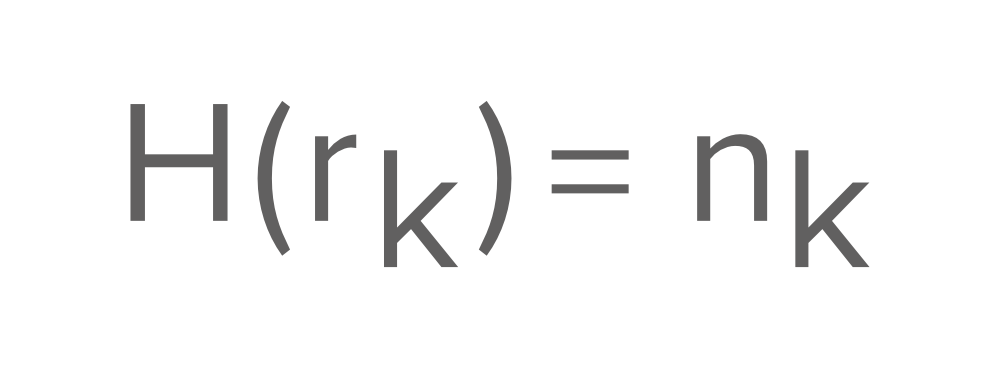Points abut Histogram:

• Histogram of an image provides a global description of the appearance of an image.
• Information botanied from histogram is very large in quality.
• Histogram of an image represents the relative frequency of occurence of various gray levels in an image.

Let’s assume that an Image matrix is given as: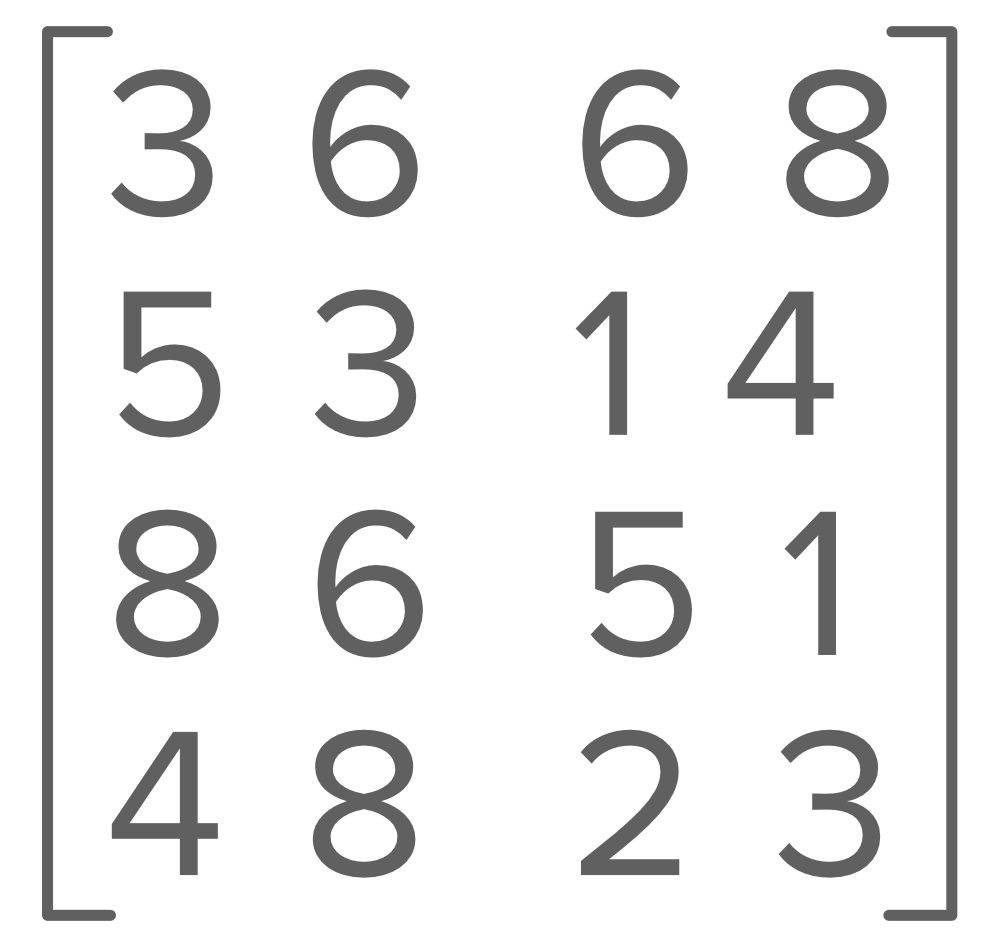This image matrix contains the pixel values at (i, j) position in the given x-y plane which is the 2D image with gray levels.

There are two ways to plot a Histogram of an image:

Method 1: In this method, the x-axis has grey levels/ Intensity values and the y-axis has the number of pixels in each grey level. The Histogram value representation of the above image is: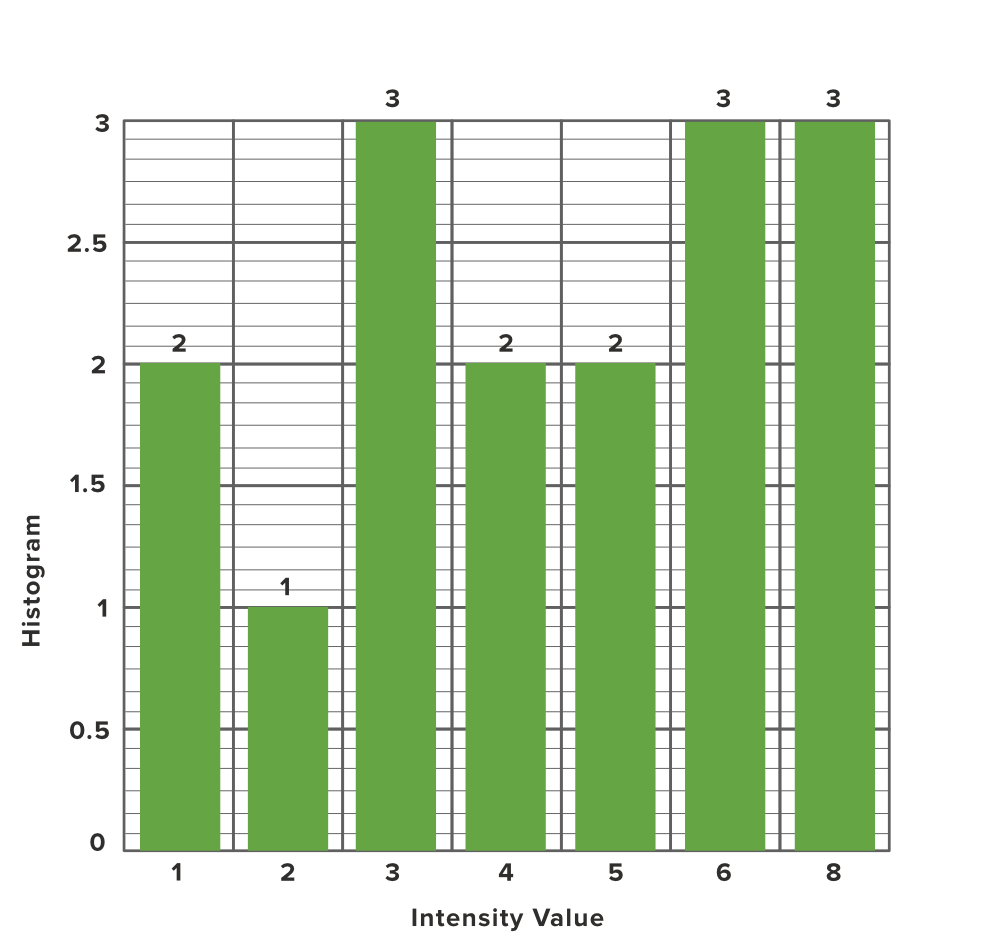Explanation: The above image has 1, 2, 3, 4, 5, 6, and 8 as the intensity values and the occurrence of each intensity value in the image matrix is 2, 1, 3, 2, 2, 3 and 3 respectively so according to intensity value and occurrence of that particular intensity we mapped them into a Graph.

Method 2: In this method, the x-axis represents the grey level, while the y-axis represents the probability of occurrence of that grey level.

Probability Function: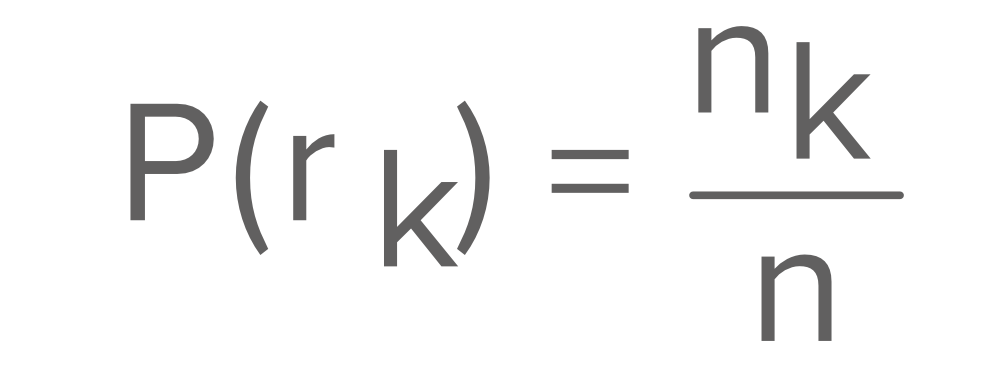Below table shows the probability of each intensity level of an pixel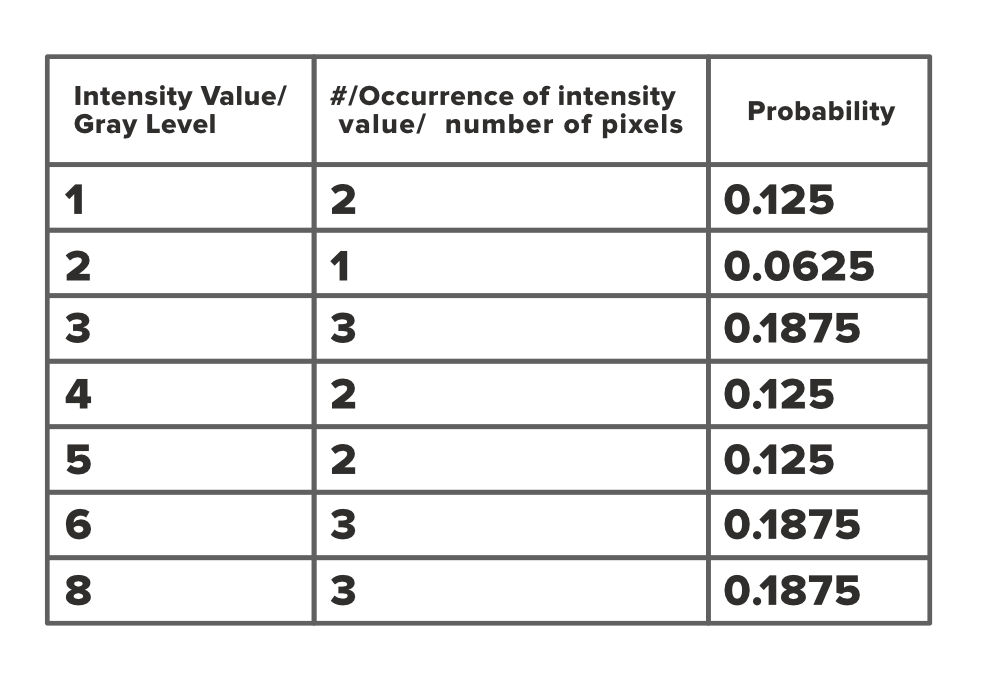Now we can create a histogram graph for each pixel and corresponding occurrence probability.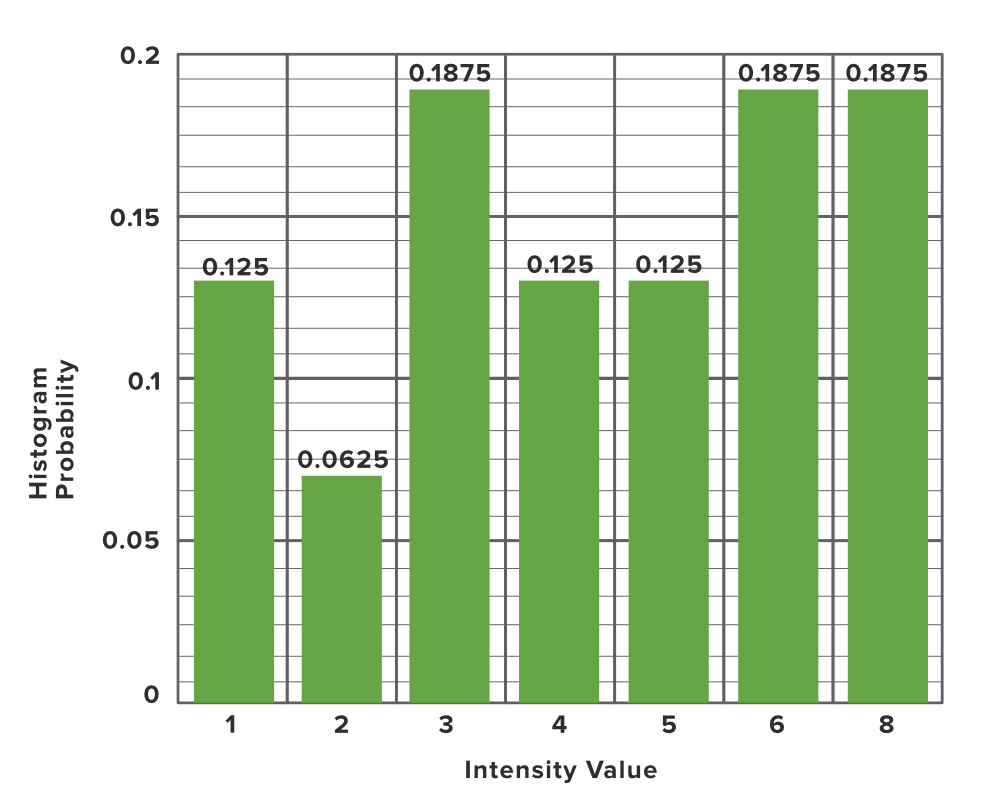My Personal Notes arrow_drop_upCheck out this Author's contributed articles.

If you like GeeksforGeeks and would like to contribute, you can also write an article using contribute.geeksforgeeks.org or mail your article to contribute@geeksforgeeks.org. See your article appearing on the GeeksforGeeks main page and help other Geeks.

Please Improve this article if you find anything incorrect by clicking on the "Improve Article" button below.